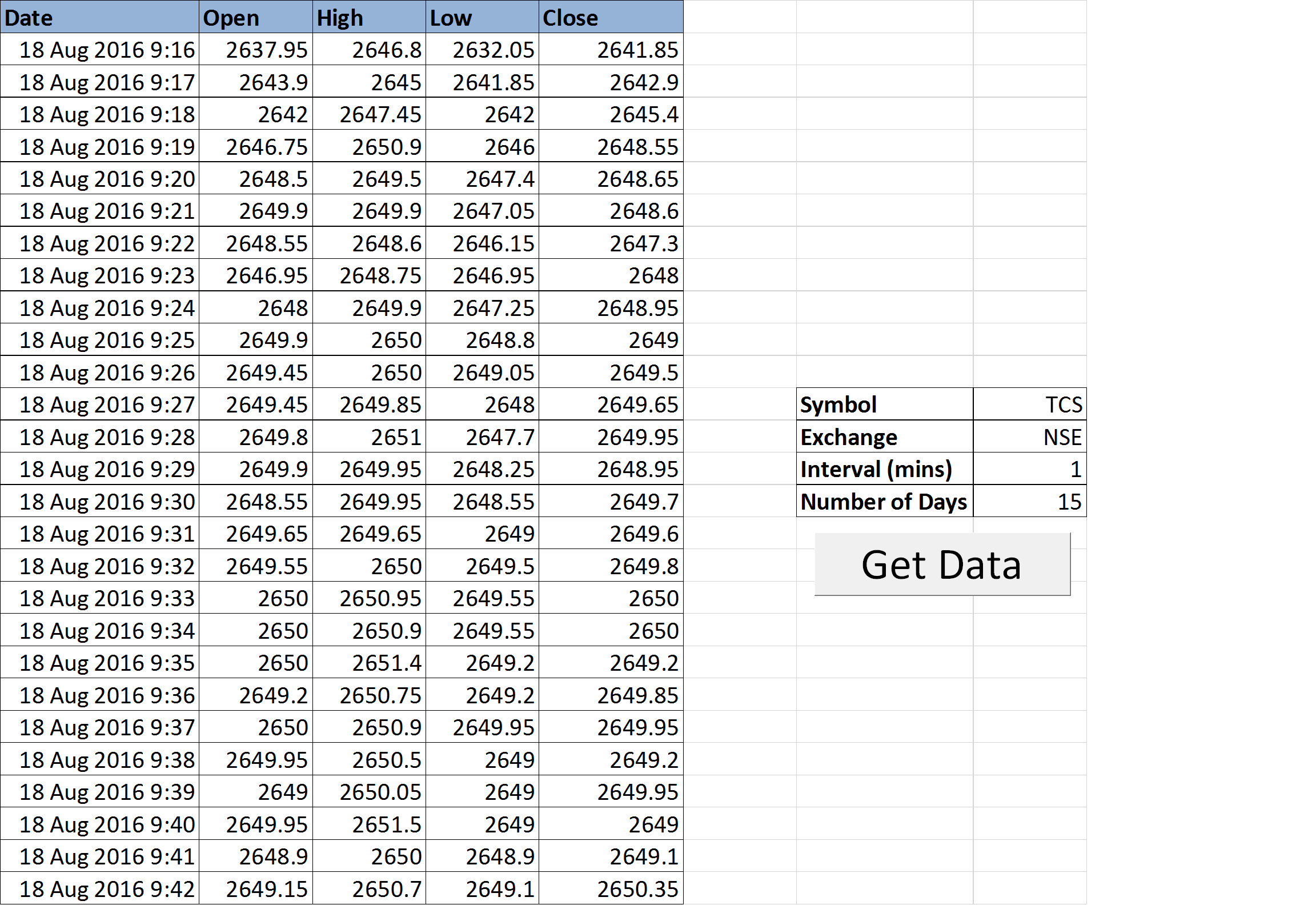Many traders prefer using Excel sheets for analyzing the stock market. However, a significant challenge arises when data needs manual copying from exchange websites or other sources to Excel. This process consumes a lot of time, which is why we’ve attempted to automate it using Excel macros and Google Finance data. You can download the automated Excel sheet at the end of this post. Having live intraday stock data in an Excel sheet can prove highly beneficial to traders who already have a buy-sell system or are looking to create one.

If you’re interested, you can also explore some of our popular trading systems that are based on Excel by clicking the link below:

### How to Utilize This Excel Sheet

Step 2: After opening the Excel file, ensure that you are connected to the internet. If prompted, please enable Macros and Data connections.

Step 3: Input the Symbol Name, Exchange Name, Interval, and Number of Days.

Step 4: Click the “Get Data” button. The data will be automatically downloaded, and the chart will refresh. Please note that this data can be downloaded for a maximum of 15 days, and the minimum interval allowed is 1 minute.

### Snapshot of Live Intraday Stock Data in the Excel Sheet:### Definition of the Macro

This Excel sheet retrieves live intraday data from Google Finance using Excel macros. You can find the macro definition below:

```				```
Option Explicit

Sub LiveData()
Dim ParameterSheet As Worksheet
Dim DataSheet As Worksheet
Dim ticker As String
Dim exchange As String
Dim interval As Integer
Dim qurl As String

Application.ScreenUpdating = False
Application.Calculation = xlCalculationManual

Set ParameterSheet = Sheets("GetData")
Set DataSheet = Sheets("Data")

DataSheet.Cells.Clear
Range("A2:E10000").Select
Selection.ClearContents
ticker = ParameterSheet.Range("symbol").Value
exchange = ParameterSheet.Range("exchange").Value
interval = ParameterSheet.Range("interval").Value * 60

"q=" & ticker & _
"&x=" & exchange & _
"&i=" & interval & _
"&p=" & numPastTradingDays & "d" & _
"&f=d,o,h,l,c,v"

QueryQuote:
.BackgroundQuery = True
.TablesOnlyFromHTML = False
.Refresh BackgroundQuery:=False
.SaveData = True
End With

DataSheet.Range("a1").CurrentRegion.TextToColumns Destination:=DataSheet.Range("a1"), DataType:=xlDelimited, _
TextQualifier:=xlDoubleQuote, ConsecutiveDelimiter:=False, Tab:=True, _
Semicolon:=False, Comma:=True, Space:=False, other:=False

DataSheet.Columns("A:G").ColumnWidth = 12

'===Convert Google timestamp to Excel timestamp (only for Windows)
Dim timeStamp As Double
Dim timeStampRaw As String
Dim timeZoneOffsetRaw As String
Dim timeZoneOffset As Variant
Dim numRows As Integer
Dim i As Integer
numRows = DataSheet.UsedRange.Rows.Count - 1

timeZoneOffsetRaw = DataSheet.Range("a7")
timeZoneOffset = (Mid(timeZoneOffsetRaw, InStr(timeZoneOffsetRaw, "=") + 1, 10))

For i = 8 To numRows

If Not IsNumeric(DataSheet.Range("a" & i)) Then

timeStampRaw = DataSheet.Range("a" & i)
timeStamp = (Mid(timeStampRaw, 2, Len(timeStampRaw) - 1))
timeStamp = (timeStamp + timeZoneOffset * 60)
DataSheet.Range("g" & i) = timeStamp / 86400 + 25569

Else

DataSheet.Range("g" & i).FormulaR1C1 = "=(RC[-6]*" & interval & "+" & timeStamp & ")/86400+25569"

End If

Next

DataSheet.Range("g8:g" & numRows).NumberFormat = "d mmm yyyy h:mm;@"
DataSheet.Range("G:G").Columns.AutoFit

'''''''''''''''''''''''''''''''''
Dim lrA As Integer

lrA = DataSheet.Range("B" & Rows.Count).End(xlUp).Row

DataSheet.Range("G8:G" & lrA).Copy
Sheet3.Range("A2").PasteSpecial Paste:=xlPasteValues
Sheet3.Range("A2:A" & lrA).NumberFormat = "d mmm yyyy h:mm;@"
Sheet3.Range("A:A").Columns.AutoFit

DataSheet.Range("E8:E" & lrA).Copy
Sheet3.Range("B2").PasteSpecial Paste:=xlPasteValues

DataSheet.Range("C8:C" & lrA).Copy
Sheet3.Range("C2").PasteSpecial Paste:=xlPasteValues

DataSheet.Range("D8:E" & lrA).Copy
Sheet3.Range("D2").PasteSpecial Paste:=xlPasteValues

DataSheet.Range("B8:B" & lrA).Copy
Sheet3.Range("E2").PasteSpecial Paste:=xlPasteValues

Application.CutCopyMode = xlCopy

'''''''''''''''''''''''''''''''''

Application.Calculation = xlCalculationAutomatic

End Sub
```
```

For the download link to access Live Intraday Stock Data in Excel Sheet, please refer to the link provided below. Most of the instructions are self-explanatory, but feel free to reach out if you have any questions. Additionally, we welcome your feedback on how we can further enhance this sheet.### The Trader’s Guide to Crude Oil Technical AnalysisdexterNovember 23, 2023### Excel-Based Moving Average Trading SystemdexterSeptember 22, 2023### Stock Alpha Calculation in a Spreadsheet: Step by Step TutorialdexterSeptember 20, 2023